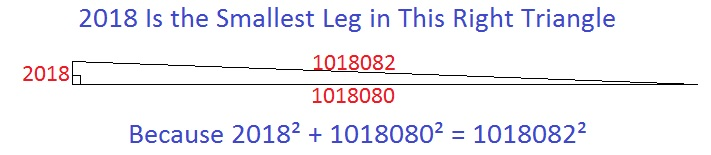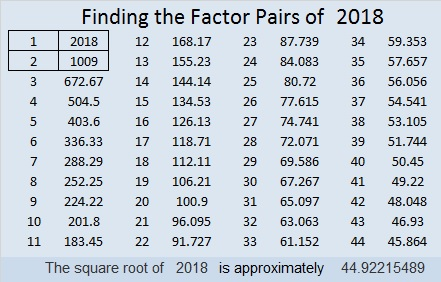# 1009, a Prime Factor of the Year 2018

Let’s begin with a mathematical equation you can use to countdown the final seconds of 2017 to welcome in the New Year, 2018.make misc GIFs like this at MakeaGif

*********

Okay, that equation had a lot of parentheses which made us multiply by 1 two different times. (Boring.) I designed it the way it is because I wanted to take advantage of the fact that 1009 × 2 = 2018.

This second equation created by Edmark M. Law needs no parentheses and is much more beautiful:make misc GIFs like this at MakeaGif

**********

Sometimes you might need a still picture instead of a gif.This post will include lots of facts about 2018.

2018¹⁰⁻⁹⁻⁸⁺⁷⁺⁶⁻⁵⁻⁴⁺³⁺²⁻¹ = 2018

2018 can be written as the sum of four consecutive numbers:
503 + 504 + 505 + 506 = 2018

It can also be written as the sum of two consecutive even numbers:
1008 + 1010 = 2018

2018 will be an amazing year in many different areas:That graphic is based on 43² +  13² = 2018.

This next one is based on the fact that 2(1009) = 2(28² +  15²) = 2018.2018 is also the sum of three squares four different ways:

36² +  19² +  19² = 2018
35² +  28² +  3² = 2018
35² +  27² +  8² = 2018
33² +  23² +  20² = 2018

This next area problem is based on one of the sums of three squares listed above. Can you tell which one?2018 is in exactly two Pythagorean triple triangles:
1118-1680-2018 and 2018-1018080-1018082.In the triangle illustrated above, 2018 is about 500 times smaller than either of the other two sides. Yep, that graphic was definitely not drawn to scale.

By contrast, in this next triangle, hypotenuse 2018 is not even twice as big as either of the legs.How did I find that triangle?
1118-1680-2018 can be calculated from 2(43)(13), 43² – 13², 43² + 13²
It is also 2 times (559-840-1009). That primitive triple can be calculated from
28² – 15², 2(28)(15), 28² + 15²

Is there any other relationship between 43² + 13² and 2(28² + 15²)? Yes.
28 + 15 = 43 and 28 – 15 = 13.

How did I find the triple with two sides in the millions (2018-1018080-1018082)?
2018 ÷ 2 = 1009 and 1009² ± 1 are the values of the other leg and the hypotenuse.

I like the way 2018 looks in these other bases:
It’s 8E8 in BASE 15 (E is 14 base 10) because 8(15²) + 14(15) + 8(1) = 2018,
2G2 in BASE 28 (16 is G base 10) because 2(28²) + 16(28) + 2(1) = 2018, and
2202202 in BASE 3 because 2(3⁶ + 3⁵ + 3³ + 3² +3⁰) = 2(1009) = 2018

This is how we can write 2018 as the sum of powers of 2:
2¹⁰ + 2⁹ + 2⁸ + 2⁷ + 2⁶ + 2⁵ + 2¹ = 2018

Finally, I give you my predictions of the factors we will see in 2018. You can be confident that these predictions will be 100% correct.
The positive factors for the year 2018 will be (drum roll) 1, 2, 1009, and 2018.
Sorry to say, but there will also be four negative factors of 2018: -1, -2, -1009, and -2018.

I also know that 2018 will have some complex factors because 43² +  13² = 2018.

Here is a graphic showing 2018’s factor pairs:Related Articles:

1. Edmark M. Law’s post titled Happy New Year 2018! (And Mathematical Facts about 2018) has many more mathematical curiosities about 2018.
2. Mathwithbaddrawings.com humorously shares some upcoming mathematical dates and other facts about 2018 in Things to Know About the Year 2018.  At least one of those facts makes the number 2018 quite unique.
3. 2018: Top Ten Facts about the New Year has a little bit of mathematics in it.

Since this is my 1009 post, I’ll tell you a few things about that number:

1009 is half of 2018.

1009 is the smallest four-digit prime number.

28² + 15² = 1009 so we get this Pythagorean triple:
559-840-1009

1009 is a palindrome or otherwise looks interesting in some other bases:
It’s 838 in BASE 11 because 8(121) + 3(11) + 8(1) = 1009,
474 in BASE 15 because 4(15²) + 7(15) + 4(1) = 1009,
321 in BASE 18 because 3(18²) + 2(18) + 1(1) = 1009,
2F2 in BASE 19 (F is 15 base 10) because 2(19²) + 15(19) + 2(1) = 1009,
1I1 in BASE 24 (I is 18 base 10) because 1(24²) + 18(24) + 1(1) = 1009, and
181 in BASE 28 because 1(28²) + 8(28) + 1(1) = 1009

• 1009 is a prime number.
• Prime factorization: 1009 is prime.
• The exponent of prime number 1009 is 1. Adding 1 to that exponent we get (1 + 1) = 2. Therefore 1009 has exactly 2 factors.
• Factors of 1009: 1, 1009
• Factor pairs: 1009 = 1 × 1009
• 1009 has no square factors that allow its square root to be simplified. √1009 ≈ 31.76476

How do we know that 1009 is a prime number? If 1009 were not a prime number, then it would be divisible by at least one prime number less than or equal to √1009 ≈ 31.8. Since 1009 cannot be divided evenly by 2, 3, 5, 7, 11, 13, 17, 19, 23, 29 or 31, we know that 1009 is a prime number.Here’s another way we know that 1009 is a prime number: Since its last two digits divided by 4 leave a remainder of 1, and 28² + 15² = 1009 with 28 and 15 having no common prime factors, 1009 will be prime unless it is divisible by a prime number Pythagorean triple hypotenuse less than or equal to √1009 ≈ 31.8. Since 1009 is not divisible by 5, 13, 17, or 29, we know that 1009 is a prime number.

Now I’ll include posts from twitter that celebrate 2018 mathematically. Some are easier to understand than others:

Be sure to click on this next one. There are MANY 2018 equations in the comments:

## 8 thoughts on “1009, a Prime Factor of the Year 2018”

1.Edmark M. Law

I found this one:

2018 = (10 × 9) + (8 × 7) – 6 + 5^4 × 3 + 2 + 1

•ivasallay

I love it. I made a gif for it and added it to the post, too. Thank you for sharing!

•Edmark M. Law

Nice:).

Can I use the gif for my New Year math post?

•ivasallay

Certainly!

2.Paula Beardell Krieg

Great timing for 1009 🙂

•ivasallay

Yes, it was. You were the only one who knew of my secret plan.

This site uses Akismet to reduce spam. Learn how your comment data is processed.